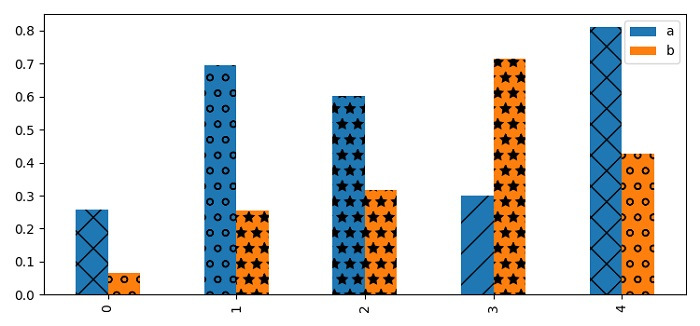# How do I plot hatched bars using Pandas and Matplotlib?

MatplotlibPythonData Visualization

To plot hatches bars using Pandas, we can take the following steps −

• Set the figure size and adjust the padding between and around the subplots.

• Make a dataframe using Pandas with two columns.

• Add an axes to the current figure as a subplot arrangement.

• Make a plot with kind="bars" class by name.

• Make a list of hatches.

• Get the bars patches using bars.patches.

• Iterate bars patches and set the hatch of each patch.

• To display the figure, use show() method.

## Example

import numpy as np
import pandas as pd
from matplotlib import pyplot as plt

plt.rcParams["figure.figsize"] = [7.50, 3.50]
plt.rcParams["figure.autolayout"] = True

df = pd.DataFrame(np.random.rand(5, 2), columns=['a', 'b'])
plt.show()